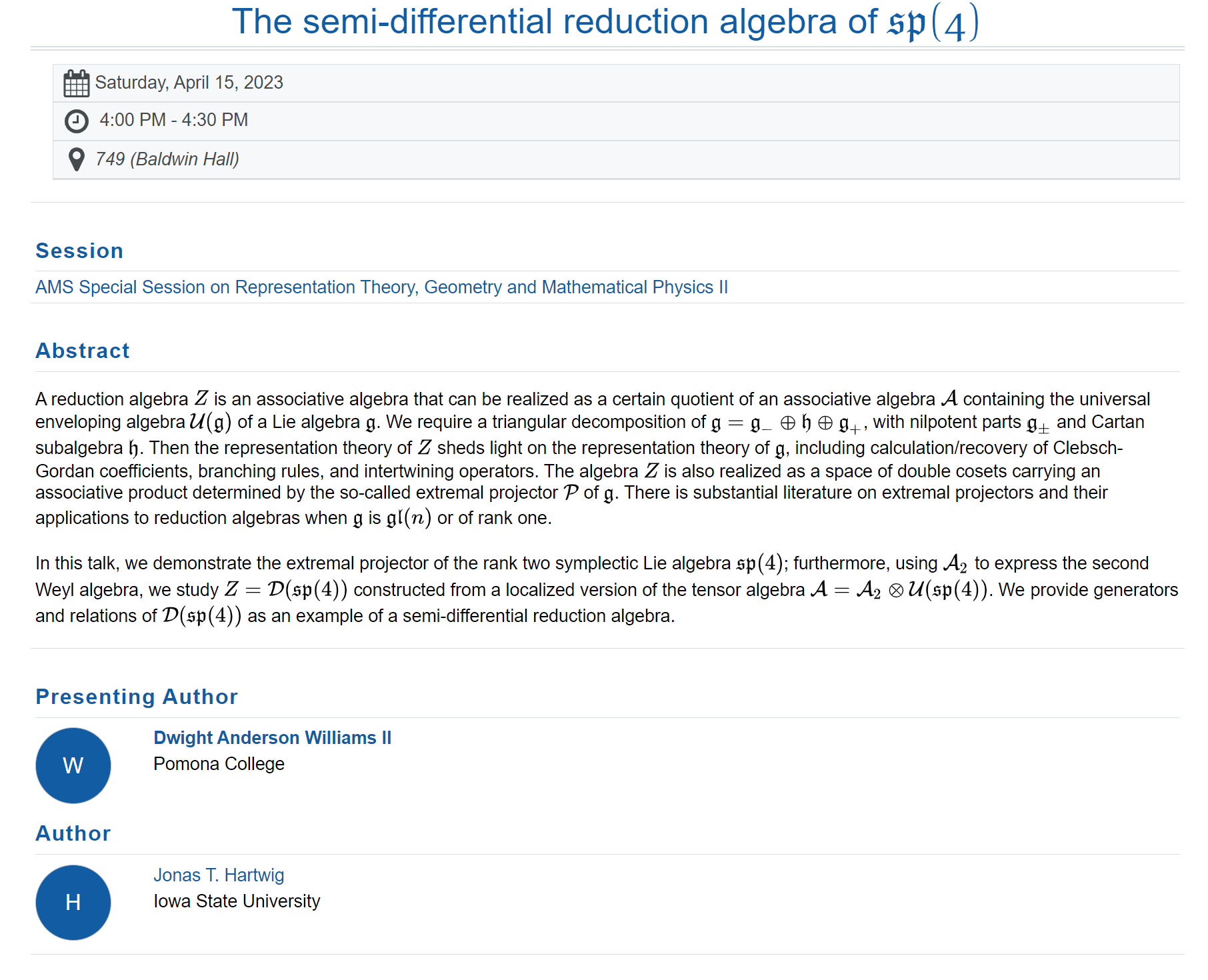# Talk: The semi-differential reduction algebra of sp(4)

Slides

Speaker: Dwight Anderson Williams II (Pomona College)

Joint Work: Jonas T. Hartwig

TItle: The semi-differential reduction algebra of $\frak{sp}(4)$

Abstract: A reduction algebra $Z$ is an associative algebra that can be realized as a certain quotient of an associative algebra $\mathcal{A}$ containing the universal enveloping algebra $\mathcal{U}(\mathfrak{g})$ of a Lie algebra $\mathfrak{g}$. We require a triangular decomposition of $$\mathfrak{g} = \mathfrak{g}_{-} \oplus \mathfrak{h} \oplus \mathfrak{g}_{+},$$ with nilpotent parts $\mathfrak{g}_{\pm}$ and Cartan subalgebra $\mathfrak{h}$. Then the representation theory of $Z$ sheds light on the representation theory of $\mathfrak{g}$, including calculation/recovery of Clebsch-Gordan coefficients, branching rules, and intertwining operators. The algebra $Z$ is also realized as a space of double cosets carrying an associative product determined by the so-called extremal projector $\mathcal{P}$ of $\mathfrak{g}$. There is substantial literature on extremal projectors and their applications to reduction algebras when $\mathfrak{g}$ is $\mathfrak{gl}(n)$ or of rank one.
In this talk, we demonstrate the extremal projector of the rank two symplectic Lie algebra $\mathfrak{sp}(4)$; furthermore, using $\mathcal{A}_{2}$ to express the second Weyl algebra, we study $$Z = \mathcal{D}(\mathfrak{sp}(4))$$ constructed from a localized version of the tensor algebra $$\mathcal{A} = \mathcal{A}_{2} \otimes \mathcal{U}(\mathfrak{sp}(4)).$$ We provide generators and relations of $\mathcal{D}(\mathfrak{sp}(4))$ as an example of a semi-differential reduction algebra.